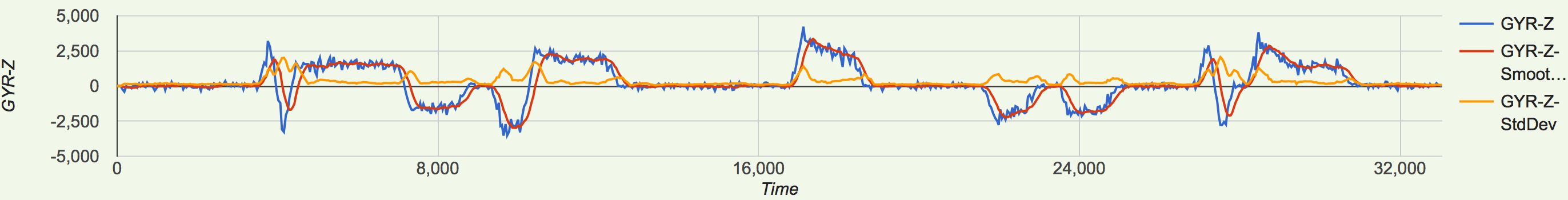## Sunday, 11. October 2015

### Introduction

After our positive experience with the data visualization, we wanted to extend it with the standard deviation of the gyro z values. It will be usefull for better detection of direction changes. And there we are with the three curves: blue are the row values, red are the moving average values and new, orange are the standard deviation values:### First track recognizer

The next step we took, was start trying to recognize curves and straights. This first attempt used only the raw data. We fixed a positive and negative threshold, and when the gyro z values are higher or lower, we have a right or a left curve, or a straight. This "silly" recognizer worked pretty good, but of course we could not trust it very much. The raw values have peaks, and these invalid all the recognition.

### Second track recognizer

After exploring the track, we want to split the track into simple parts. For storing the data we implemented a simple model with a TrackPart class, and an enum for the direction of the track-part:

``````public class TrackPart {

private Direction direction;
...
}

public enum Direction {

RIGHT, LEFT, STRAIGHT

}``````

This "enhanced" curve recognizer uses moving averages. So we avoid peak values and use the nice red function in our graphs. The mechanism is again very simple: we read the values, and when they reach certain thresholds, we create a new instance of the TrackPart class in which we save the direction, start and end times. When the values reach another threshold, we create again the corresponding instance.

We also use the standard deviation to avoid that we detect a STRAIGHT-Part between every curve.

We save all these instances in a Track container class, because what we want is...

### Periodicity

Yes, we want to know when a new lap starts. In order to achieve that, starting from all the saved TrackPart instances we create a pattern like: RIGHT - LEFT - RIGHT -RIGHT - LEFT - STRAIGHT - LEFT. See an example:

``````TrackPart[direction=STRAIGHT, start=0, end=3677]
TrackPart[direction=RIGHT, start=3677, end=4238]
TrackPart[direction=LEFT, start=4238, end=4666]
TrackPart[direction=RIGHT, start=4666, end=7469]
TrackPart[direction=LEFT, start=7469, end=8966]
TrackPart[direction=STRAIGHT, start=8966, end=9598]
TrackPart[direction=LEFT, start=9598, end=10568]
TrackPart[direction=RIGHT, start=10568, end=12727]
TrackPart[direction=STRAIGHT, start=12727, end=16945]
TrackPart[direction=RIGHT, start=16945, end=18936]
TrackPart[direction=STRAIGHT, start=18936, end=21628]
TrackPart[direction=LEFT, start=21628, end=23158]
TrackPart[direction=STRAIGHT, start=23158, end=23636]
TrackPart[direction=LEFT, start=23636, end=25195]
TrackPart[direction=STRAIGHT, start=25195, end=27195]
TrackPart[direction=RIGHT, start=27195, end=27726]
TrackPart[direction=LEFT, start=27726, end=28245]``````

After each recognized TrackPart we split the list into two same size lists. We compare them and check if it's the same pattern. Thats how we get candidates for our track pattern. After the second round we will get a match which is our track pattern. To detect the best match we use the round-time-event. If we add up all the times of our trackparts, the difference to the round-time should be as small as possible.

After we recognized the track we have to implement a logic which detects our position in the track at any time. Still we have to check our logic, and of course, test all.

Thats all, folks...